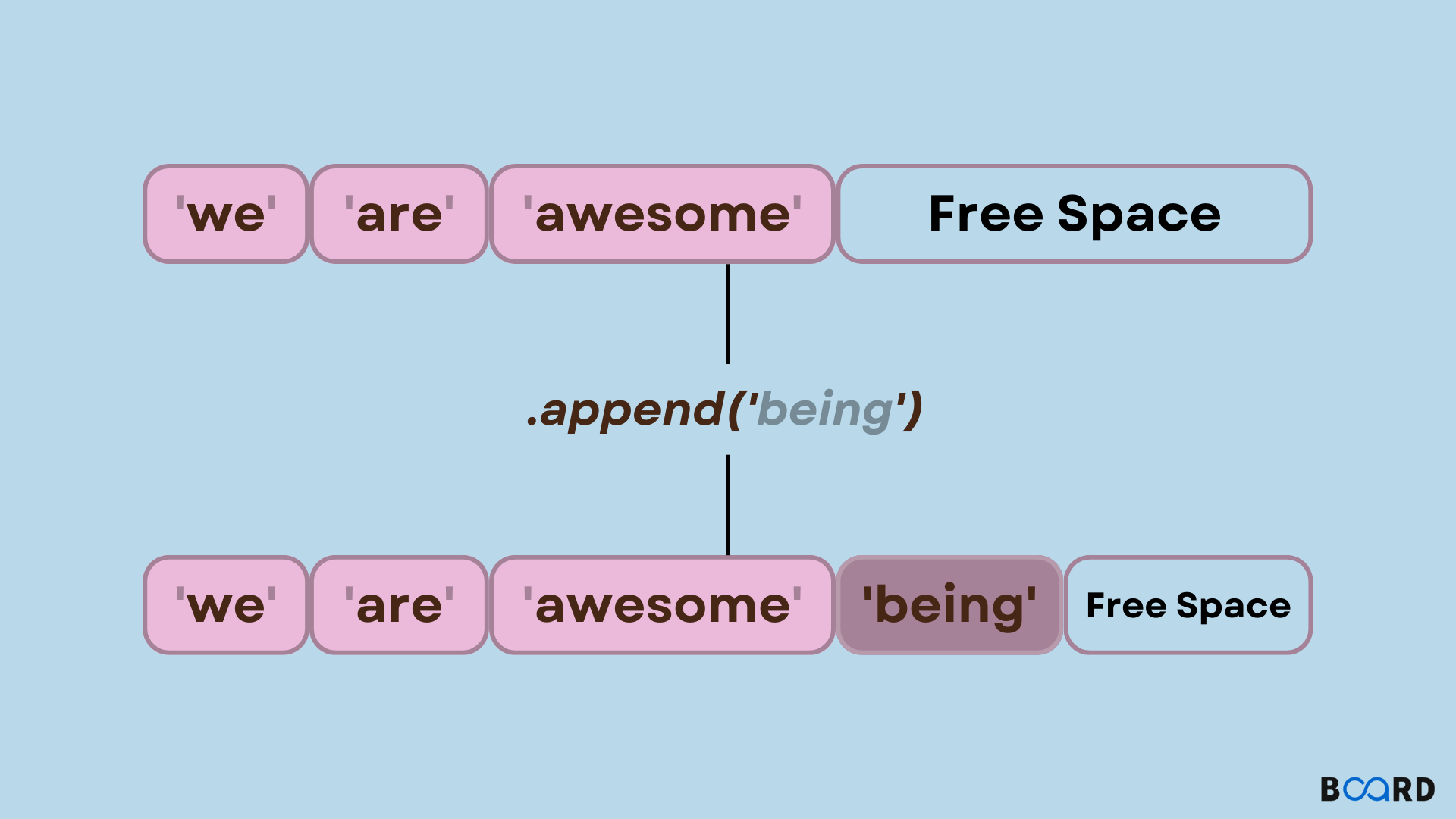# Python List append() Method## Introduction

In Python, if a user wishes to add elements at the end of the list, then there is a method named append() with which it can be achieved.

Syntax

 list.append(item)

append() Parameters

This method takes a single parameter.

item: This item can be a number, string, or even a list itself etc. This item is added at the end of the list.

Return Value from append()

This method returns none. That means this method returns nothing.

## Examples

### Example 1: Appending an Item to the List

Code

 # list named ll = ['A', 'B']  l.append('C') # appending 'C' to the list l  print l

Output

 ['A', 'B', 'C']
write your code here: Coding Playground

### Example 2: Appending a List to the List

Code

 # list named l1l1 = ['A', 'B', 'C']  # another list named l2l2 = [1, 2, 3]  l1.append(l2) # appending l2 to l1  print l1

Output

 ['A', 'B', 'C', [1, 2, 3]]
write your code here: Coding Playground

## append() method in other Data Structures

In Python append(0 methods can also be implemented on other data structures. The working principle is the same as the list. The method does is that it adds a single element to the end of the data structure, but there are some minor differences.

### array.append()

In Python, an array is a sequential data structure that can compactly store a sequence of values. These are similar to the list but there is one slight condition that is the items stored in the array must be of the same data type that is limited to C-style data types such as integer numbers, characters, floating numbers etc.

In Python, array.array() provides the array data structure and it takes two arguments.

• typecode: This is a single-character code that signifies the data type of the items that can be stored in an array. This argument is compulsory.
• initializer: It can be an iterable, bytes-like object or a list that is used as an initializer.

Code

 from array import arrayarr = array("i", [1,2,3,4])print(arr)print(arr)print(arr[:2])arr = 8print(arr)

Output

 array('i', [1, 2, 3, 4])2array('i', [1, 2])array('i', [1, 2, 8, 4])

In arrays, we can use append to add an element at the last of the array.

Code

 from array import arrayarr = array("i", [1,2,3,4])arr.append(5)print(arr)

Output

 array('i', [1, 2, 3, 4, 5])

But if we try to append an element of a different data type then it will raise an error.

Code

 from array import arrayarr = array("i", [1,2,3,4])arr.append(5.0)print(arr)

Output

 Traceback (most recent call last):  File "main.py", line 3, in     arr.append(5.0)TypeError: integer argument expected, got float

But if we try to append an integer to an array storing float data type elements, then Python will automatically convert the integer data type element to a float data type element.

Code

 from array import arrayarr = array("f", [1.0, 2.0, 3.0, 4.0])arr.append(5)print(arr)

Output

 array('f', [1.0, 2.0, 3.0, 4.0, 5.0])
write your code here: Coding Playground